## In the figure, ABCDE is a pentagon with BE ∥ CD and BC ∥ DE. BC is perpendicular to CD. AB = 5cm, AE = 5cm, BE = 7cm, BC =  x − y and CD =  x + y. If the perimeter of ABCDE is 27 cm. find the value of x and y, given x, y ≠ 0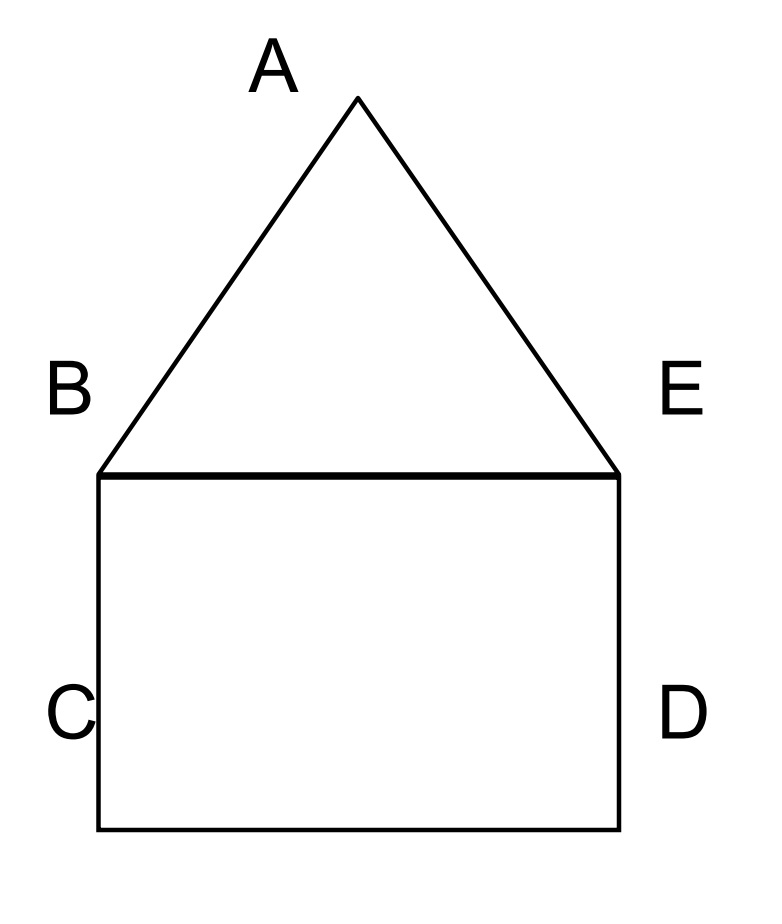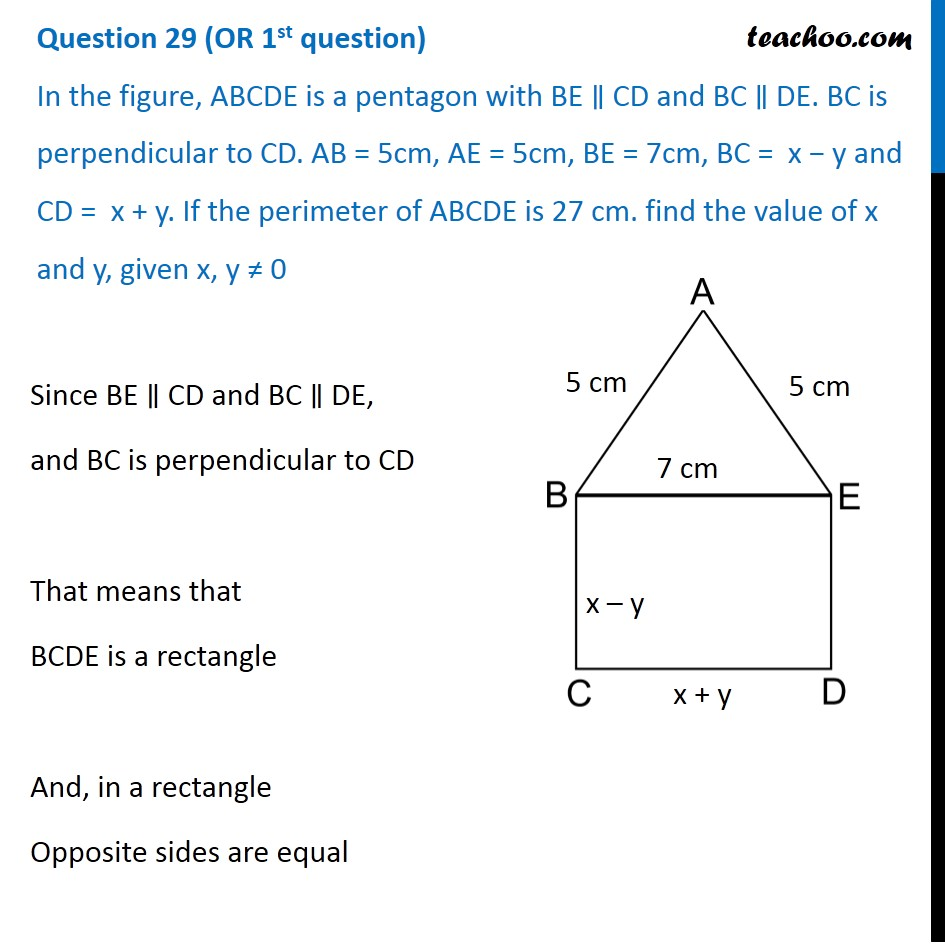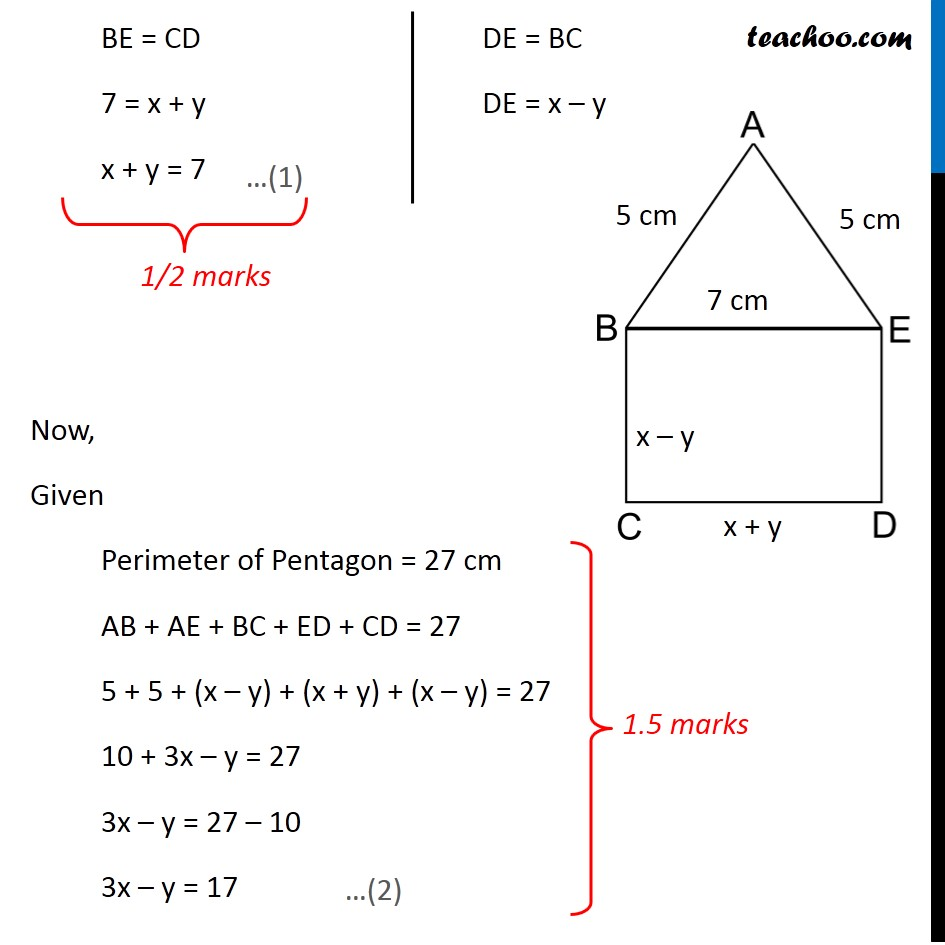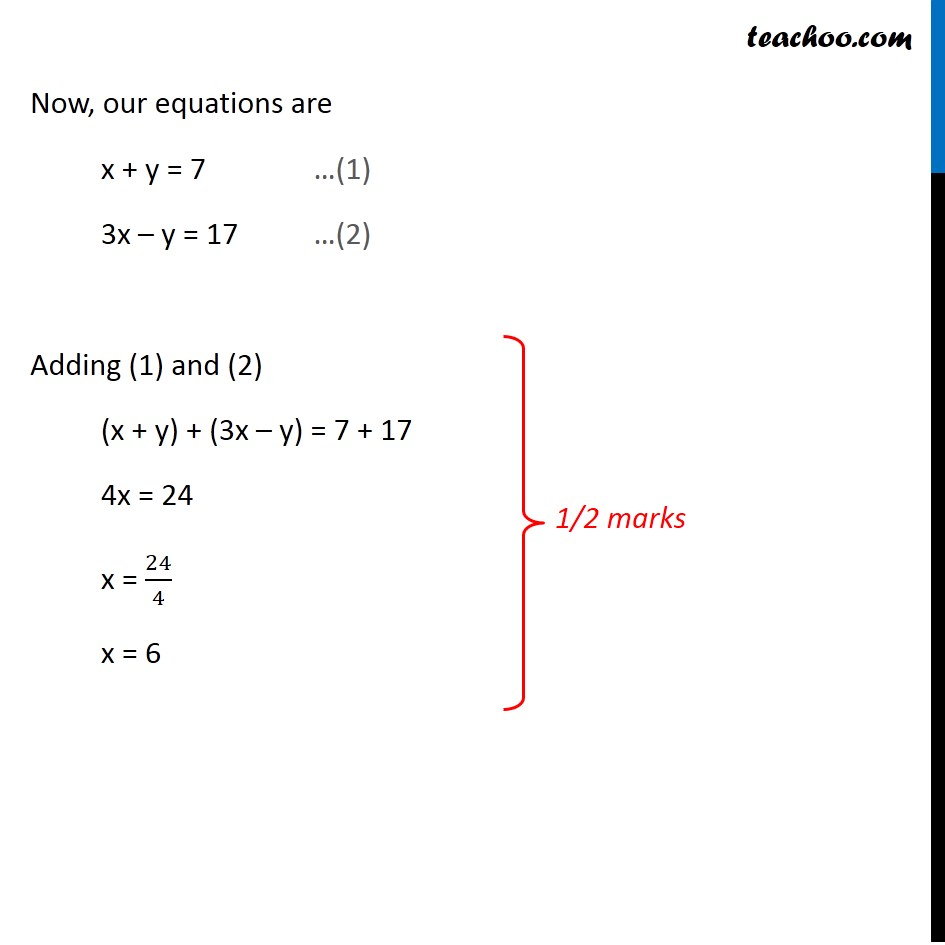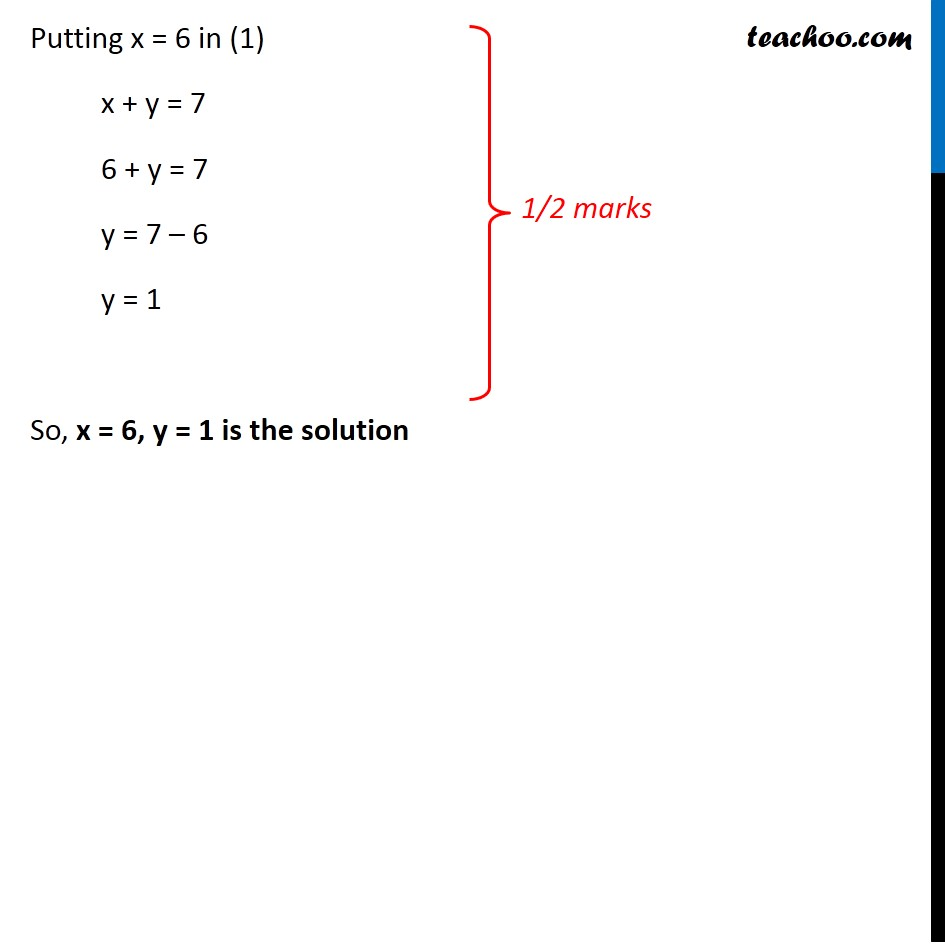Subscribe to our Youtube Channel - https://you.tube/teachoo

1. Class 10
2. Solutions of Sample Papers for Class 10 Boards
3. CBSE Class 10 Sample Paper for 2020 Boards - Maths Standard

Transcript

Question 29 (OR 1st question) In the figure, ABCDE is a pentagon with BE ∥ CD and BC ∥ DE. BC is perpendicular to CD. AB = 5cm, AE = 5cm, BE = 7cm, BC = x − y and CD = x + y. If the perimeter of ABCDE is 27 cm. find the value of x and y, given x, y ≠ 0 Since BE ∥ CD and BC ∥ DE, and BC is perpendicular to CD That means that BCDE is a rectangle And, in a rectangle Opposite sides are equal BE = CD 7 = x + y x + y = 7 Now, Given Perimeter of Pentagon = 27 cm AB + AE + BC + ED + CD = 27 5 + 5 + (x – y) + (x + y) + (x – y) = 27 10 + 3x – y = 27 3x – y = 27 – 10 3x – y = 17 Now, our equations are x + y = 7 …(1) 3x – y = 17 …(2) Adding (1) and (2) (x + y) + (3x – y) = 7 + 17 4x = 24 x = 24/4 x = 6 Putting x = 6 in (1) x + y = 7 6 + y = 7 y = 7 – 6 y = 1 So, x = 6, y = 1 is the solution

CBSE Class 10 Sample Paper for 2020 Boards - Maths Standard

Class 10
Solutions of Sample Papers for Class 10 Boards